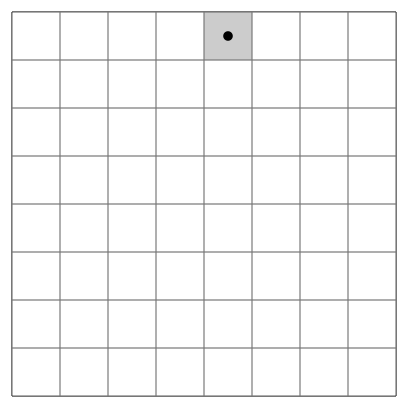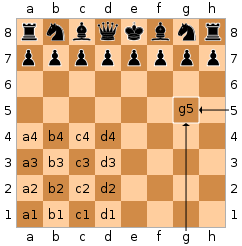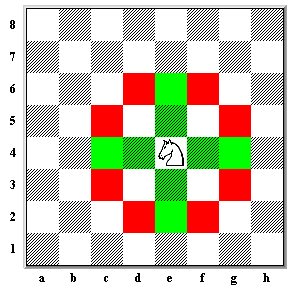In this post, let’s solve the “Euler Knight’s Tour” using F#.

## Disclaimer

Sometimes life imposes a pause on us. I’m going through one of these moments now.

Kindly, I will take a little time to write some code without worrying about quality standards or practical results. That is the case about the code I am sharing today. It was written just for fun.

## Euler Knight’s Tour?

From wikipedia:

knight’s tour is a sequence of moves of a knight on a chessboard such that the knight visits every square only once. If the knight ends on a square that is one knight’s move from the beginning square (so that it could tour the board again immediately, following the same path), the tour is closed, otherwise it is open.

The knight’s tour problem is the mathematical problem of finding a knight’s tour. Creating a program to find a knight’s tour is a common problem given to computer science students. Variations of the knight’s tour problem involve chessboards of different sizes than the usual 8 × 8, as well as irregular (non-rectangular) boards.

The following animation is an example of a knight’s tour:## Chessboard coordinates

Before working on the Knight’s Tour, we need to understand and write code about some core chess concepts. Let’s start with chess coordinates.

From wikipedia:

Each square of the chessboard is identified by a unique coordinate pair—a letter and a number. The vertical columns of squares, called files, are labeled a through hfrom White’s left (the queenside) to right (the kingside). The horizontal rows of squares, called ranks, are numbered 1 to 8 starting from White’s side of the board. Thus each square has a unique identification of file letter followed by rank number. (For example, White’s king starts the game on square e1; Black’s knight on b8 can move to open squares a6 or c6.)Did you get it? Additionally, I am associating a number for each square. Starting with 0 for a1, 1 for b1, 2 for c1, until 63 for h8.

Here is the code that deals with chessboard coordinates:

```let si2fi squareIndex =
(squareIndex % 8)

let file squareIndex =
'a' + (char (si2fi squareIndex))

file 0  // a
file 1  // b
file 8  // c
file 63 // h

let si2ri squareIndex =
(int (squareIndex / 8))

let rank squareIndex =
(si2ri squareIndex) + 1

rank 0  // 1
rank 1  // 1
rank 8  // 2
rank 63 // 8

let identifier squareIndex =
sprintf "%c%d" (file squareIndex) (rank squareIndex)

identifier 0   // a1
identifier 1   // b1
identifier 8   // a2
identifier 63  // h8

let identifier2ri (identifier:string) =
int(identifier.) - int('1')

let identifier2fi (identifier:string) =
int(identifier.) - int ('a')

let si ri fi =
ri * 8 + fi

let identifier2si (identifier:string) =
si (identifier2ri identifier) (identifier2fi identifier)

identifier2si "a1"  // 0
identifier2si "b1"  // 1
identifier2si "a2"  // 8
identifier2si "h8"  // 63
```

As you can see, I did not implement any validation. In a “real-world” scenario, I would use option as return type for almost all these functions.

## The Knight Move

From wikipedia:

The knight move is unusual among chess pieces. It moves to a square that is two squares away horizontally and one square vertically, or two squares vertically and one square horizontally. The complete move therefore looks like the letter “L”.Here is my code to generate a list of movements from a specified square:

```let moves from =
let r = si2ri from
let f = si2fi from
seq {
if r >= 2 then
if f > 0 then yield (si (r - 2) (f - 1))
if f < 7 then yield (si (r - 2) (f + 1)) if r >= 1 then
if f > 1 then yield (si (r - 1) (f - 2))
if f < 6 then yield (si (r - 1) (f + 2))
if r <= 5 then if f > 0 then yield (si (r + 2) (f - 1))
if f < 7 then yield (si (r + 2) (f + 1))
if r <= 6 then if f > 1 then yield (si (r + 1) (f - 2))
if f < 6 then yield (si (r + 1) (f + 2)) } moves (identifier2si "c1") |> Seq.map identifier
|> Seq.iter (printf "%A ") // "b3" "d3" "a2" "e2"

moves (identifier2si "a1")
|> Seq.map identifier
|> Seq.iter (printf "%A ") // "b3" "c2"

moves (identifier2si "h1")
|> Seq.map identifier
|> Seq.iter (printf "%A ") // "g3" "f2"

moves (identifier2si "h8")
|> Seq.map identifier
|> Seq.iter (printf "%A ") // "g6" "f7"

moves (identifier2si "e5")
|> Seq.map identifier
|> Seq.iter (printf "%A ") // "d3" "f3" "c4" "g4" "d7" "f7" "g6" "c6"
```

## The Bitboard

From wikipedia:

A bitboard, often used for boardgames such as chess, checkers, othello and word games, is a specialization of the bit array data structure, where each bit represents a game position or state, designed for optimization of speed and/or memory or disk use in mass calculations. Bits in the same bitboard relate to each other in the rules of the game, often forming a game position when taken together. Other bitboards are commonly used as masks to transform or answer queries about positions. The “game” may be any game-like system where information is tightly packed in a structured form with “rules” affecting how the individual units or pieces relate.

I decided to use bitboards to store which squares were visited by the knight.

```let emptyBoard = uint64(0)

let si2bit (si) =
uint64(1) <<< si

si2bit 0  // 1
si2bit 1  // 2
si2bit 2  // 4

let isClear board si =
(board &&& (si2bit si)) = uint64(0)

let set board si =
(board ||| si2bit(si), si)

let possibleMoves board from =
moves from
|> Seq.where (isClear board)

```

The ‘possibleMoves’ function filters the list of moves from a source square ignoring target squares that were already visited.

## Making Moves

We are ready to start moving the knight through the board.

```let makeMove moveselector board from =
let alternatives = possibleMoves board from |> List.ofSeq
if (Seq.isEmpty alternatives)
then None
else alternatives
|> moveselector board
|> set board
|> Some

let first board alternatives =

makeMove first emptyBoard (identifier2si "a1")

let go moveselector from = seq {
let rec innerGo moveselector board from = seq {
let result = makeMove moveselector board from
match result with
| Some(newboard, selectedmove) ->
yield selectedmove
yield! innerGo moveselector newboard selectedmove
| None -> ()
}

let (board, _) = set emptyBoard from
yield from
yield! innerGo moveselector board from
}

go first (identifier2si "a1")
|> Seq.map (identifier)
|> Seq.iter (printfn "%A")
```

My first “naïve” attempt to solve this puzzle was to pick the first possible move from the last visited (or start) square, ignoring already visited squares, and make it. Then I repeat this process in the resulting position until it is not possible.

## Rendering the result

We are moving the knight and it would be nice to have some kind of visual representation. Am I right? Let’s do it.

```let history moves =
let consolidated = moves |> Array.ofSeq
Array.init 64 (fun x -> if (Array.exists ((=) x) consolidated)
then Some(Array.findIndex ((=) x) consolidated)
else None
)

identifier2si "a1"
|> go first
|> history

let render (history : int option []) =
let isNewLine index =
((index + 1) % 8 = 0)

for index in 63..-1..0 do

if isNewLine index then
printfn ""
if (index > 0) then printf "|"

if (index >= 0) then
let basei = int(index / 8) * 8
let worki = (7 - (index - basei)) + basei
match history.[worki] with
| Some(step) -> printf "%2i|" step
| _ -> printf "  |"

printfn ""

identifier2si "h8"
|> go first
|> history
|> render
```

We start filling an array with 64 positions, each one indicating one square from the board. The value is the movement number or none.

The ‘render’ function prints a textual representation of the board with numbers indicating the sequence of movements.

```|  |  |  |  |  |  |  |  |
|  |  |  |  |  |  |  |  |
|  |  |  |  |  |  |  |  |
|  |  |  |  |  |  |  |  |
|  |14|  |  |  |  |  |  |
|12| 1|10| 3|  | 5|  | 7|
|15|  |13|  |  | 8|  |  |
| 0|11| 2| 9| 4|  | 6|  |
```

Obviously, making the first possible move is not a good idea. Let’s adopt another strategy: The Warnsdorf’s rule.

From wikipedia:

Warnsdorf’s rule is a heuristic for finding a knight’s tour. The knight is moved so that it always proceeds to the square from which the knight will have the fewest onward moves. When calculating the number of onward moves for each candidate square, we do not count moves that revisit any square already visited.

My implementation:

```let lessAccessible board alternatives =
let possibleMovesCount from =
possibleMoves board from
|> Seq.length

alternatives
|> List.map (fun a -> (a, possibleMovesCount a))
|> List.minBy (fun (a, c) -> c)
|> fst

identifier2si "a1"
|> go lessAccessible
|> history
|> render

for si in 0..63 do
let result = go lessAccessible si |> Seq.length
if (result <> 64) then
printfn "Failed with %A..." (identifier si)
```

Now are tour, starting from “a1” is complete.

```|43| 6|41|58|45| 8|51|62|
|40|57|44| 7|52|61|36| 9|
| 5|42|59|54|37|46|63|50|
|56|39|26|47|60|53|10|35|
|19| 4|55|38|27|34|49|32|
|22| 1|20|25|48|31|14|11|
| 3|18|23|28|13|16|33|30|
| 0|21| 2|17|24|29|12|15|
```

But, we are failing when starting from “f8”

```|13|10|29|52|15| 0|33|58|
|28|41|14|11|  |59|16| 1|
| 9|12|51|30|53|32|57|34|
|40|27|42|  |48|  | 2|17|
|23| 8|39|50|31|54|35|56|
|26|43|24|47|  |49|18| 3|
| 7|22|45|38| 5|20|55|36|
|44|25| 6|21|46|37| 4|19|
```

## Breaking Ties

It is, of course, possible to have two or more choices for which the number of onward moves is equal – that is probably the cause we are failing to complete the tour starting from “f8”. There are various methods for breaking such ties but I decided to adopt something simple.

```let possibleMovesCount board from =
possibleMoves board from
|> Seq.length

let lessAccessible2 board alternatives =
let nextStepPossibleMovesCount m =
let b = set board m |> fst

let nextMoves = possibleMoves b m |> Array.ofSeq
if Array.isEmpty(nextMoves)
then 1000
else
nextMoves
|> Seq.map (fun m -> possibleMovesCount b m)
|> Seq.min

let weight move =
(possibleMovesCount board move) * 10 + (nextStepPossibleMovesCount move)

alternatives
|> Seq.map (fun move -> (move, weight move))
|> Seq.minBy (fun (_, weight) -> weight)
|> fst

identifier2si "f8"
|> go lessAccessible2
|> history
|> render
```

Adopting this strategy, we are ready to complete the tour even starting from “f8”

```|13|10|29|52|15| 0|31|36|
|28|51|14|11|30|37|16| 1|
| 9|12|53|46|63|32|35|38|
|50|27|62|33|54|45| 2|17|
|23| 8|49|58|47|34|39|44|
|26|61|24|55|42|57|18| 3|
| 7|22|59|48| 5|20|43|40|
|60|25| 6|21|56|41| 4|19|
```

## The work is done!

We successfully implemented a solver for the Euler Knight’s Tour using F#. The code is not perfect, but works. Anyway, I am open to feedback. Let’s make it better?